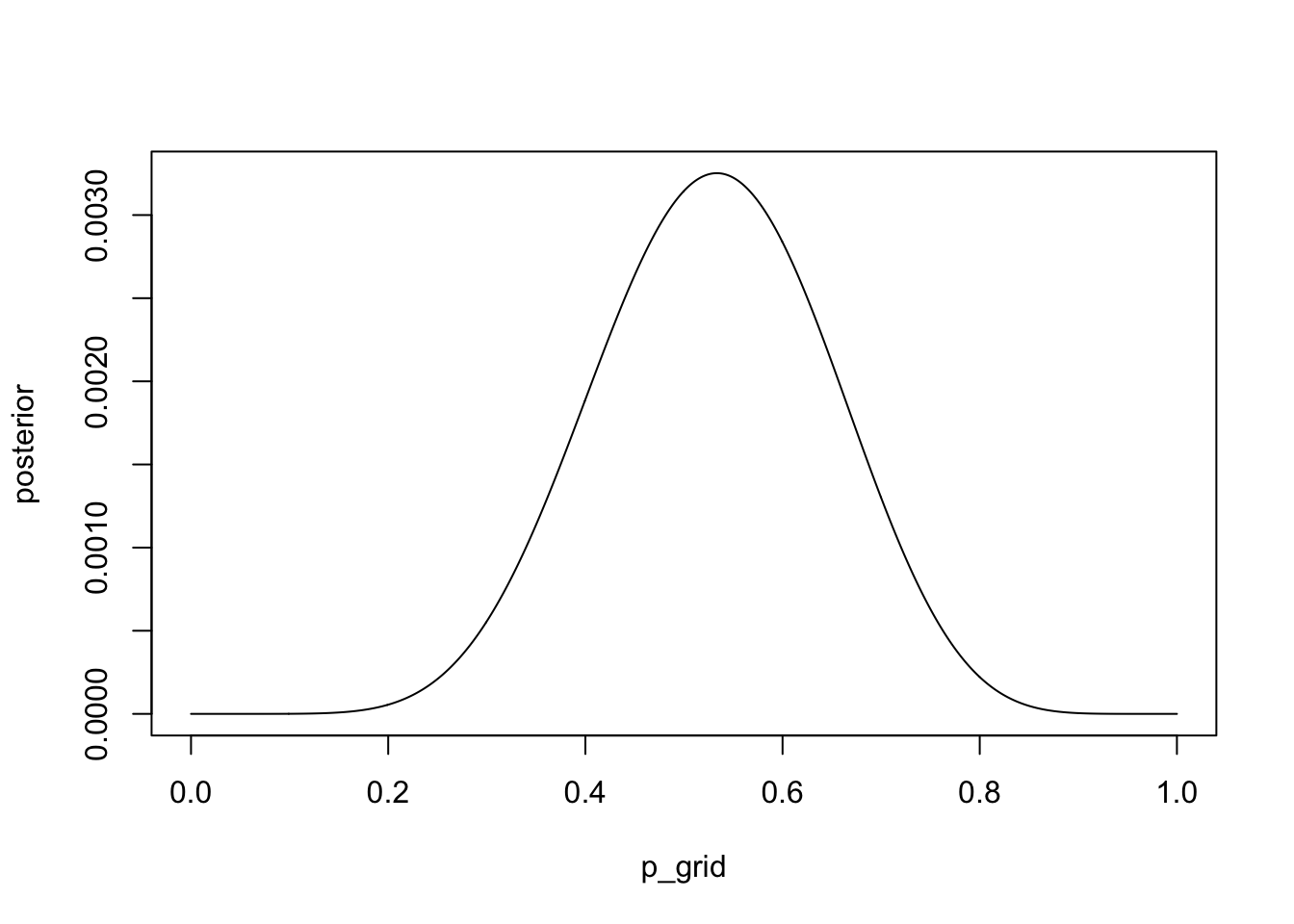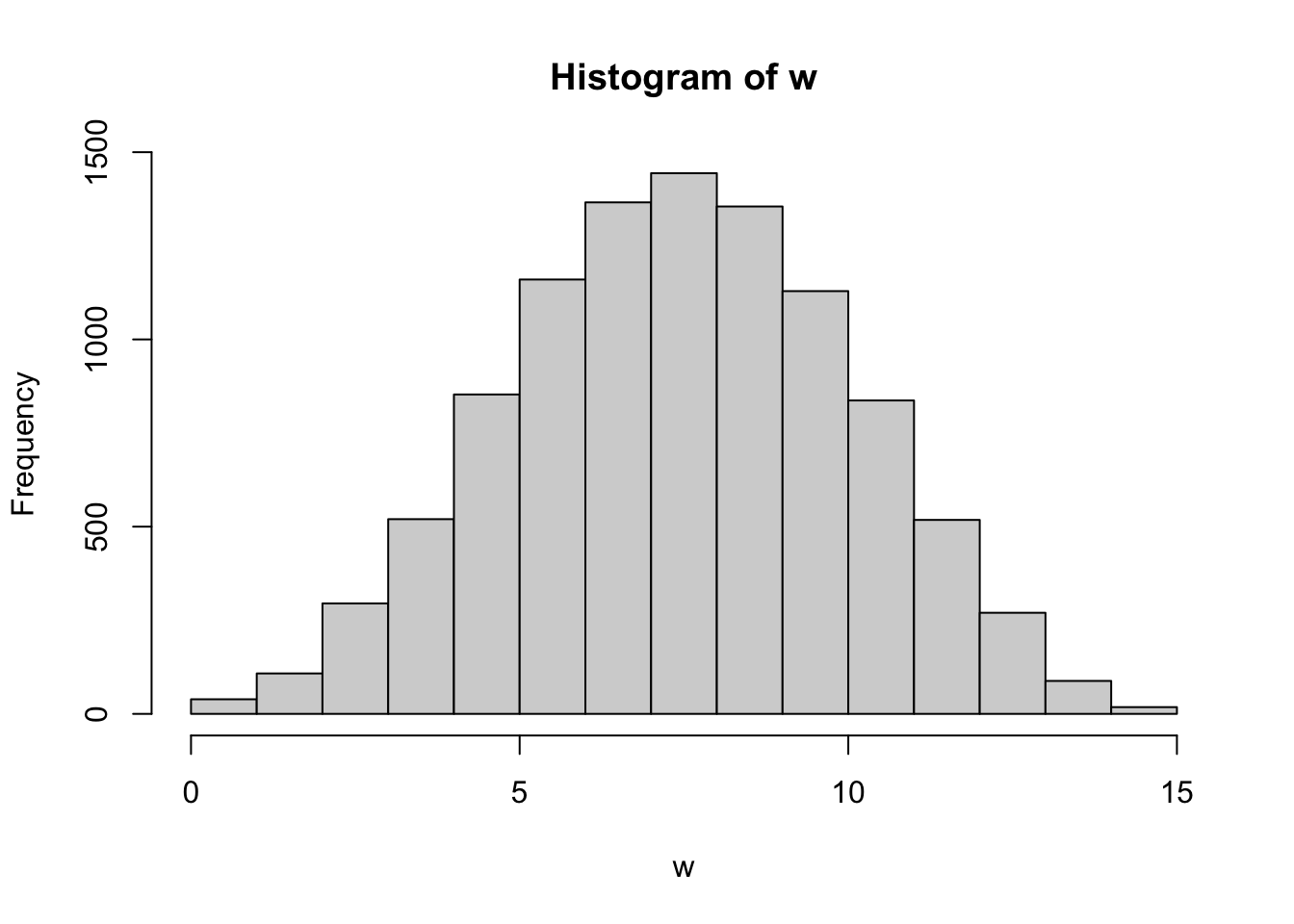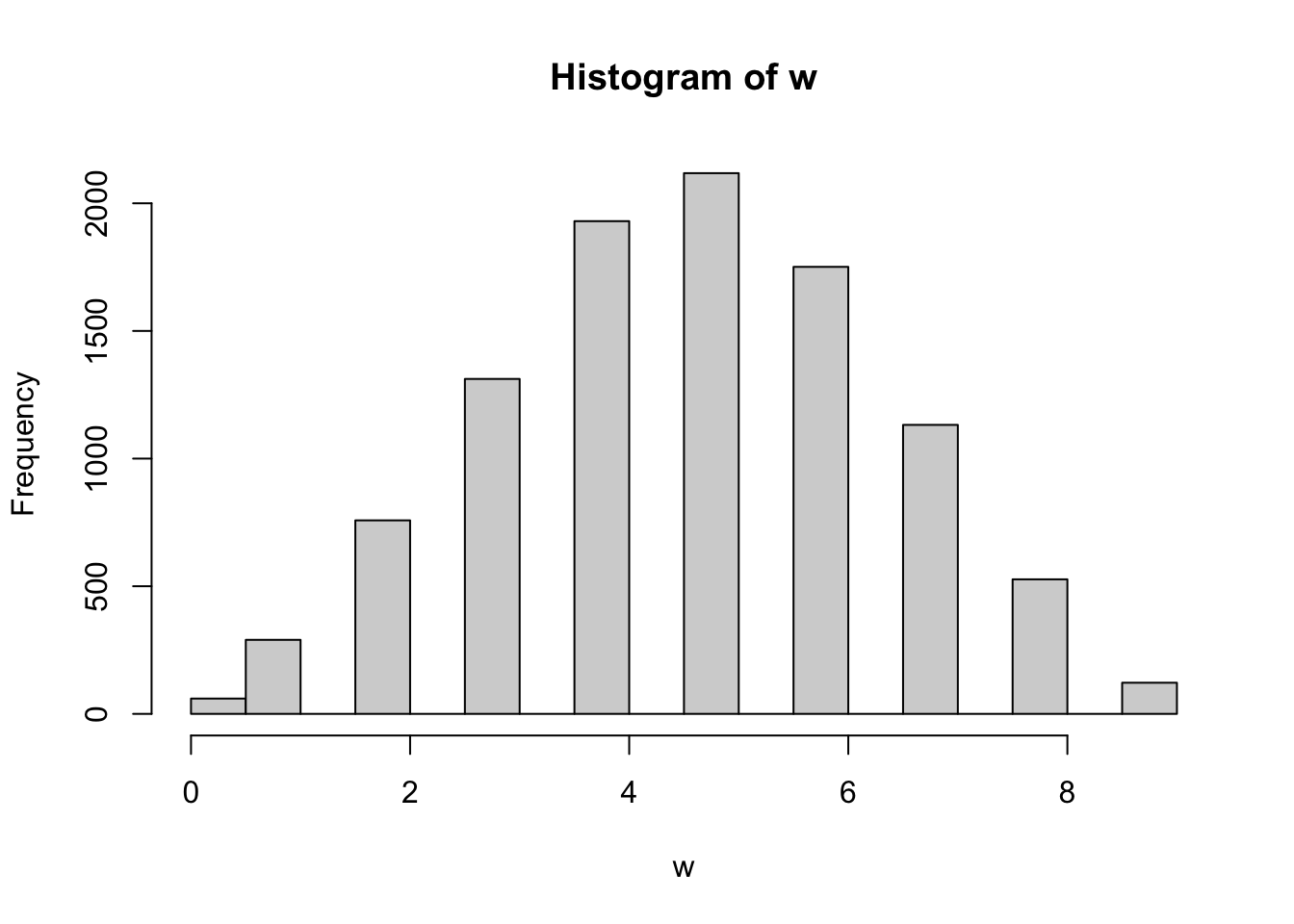## Chapter 3 - Sampling the Imaginary

This chapter introduced the basic procedures for manipulating posterior distributions. Our fundamental tool is samples of parameter values drawn from the posterior distribution. These samples can be used to produce intervals, point estimates, posterior predictive checks, as well as other kinds of simulations. Posterior predictive checks combine uncertainty about parameters, as described by the posterior distribution, with uncertainty about outcomes, as described by the assumed likelihood function. These checks are useful for verifying that your software worked correctly. They are also useful for prospecting for ways in which your models are inadequate.

Place each answer inside the code chunk (grey box). The code chunks should contain a text response or a code that completes/answers the question or activity requested. Make sure to include plots if the question requests them. Problems are labeled Easy (E), Medium (M), and Hard(H).

Finally, upon completion, name your final output `.html` file as: `YourName_ANLY505-Year-Semester.html` and publish the assignment to your R Pubs account and submit the link to Canvas. Each question is worth 5 points

## Simulate the posterior distribution

``````p_grid <- seq( from=0 , to=1 , length.out=1000 )
prior <- rep( 1 , 1000 )
likelihood <- dbinom( 6 , size=9 , prob=p_grid )
posterior <- likelihood * prior
posterior <- posterior / sum(posterior)
set.seed(100)
samples <- sample( p_grid , prob=posterior , size=1e4 , replace=TRUE )``````

## Questions

Use the values in samples to answer the questions that follow.

3E1. How much posterior probability lies below p = 0.2?

``sum(samples < 0.2) / 1e4``
``##  4e-04``
``mean(samples < 0.2)``
``##  4e-04``

3E2. How much posterior probability lies above p = 0.8?

``mean(samples > 0.8)``
``##  0.1116``

3E3. How much posterior probability lies between p = 0.2 and p = 0.8?

``mean(samples > 0.2 & samples < 0.8)``
``##  0.888``

3E4. 20% of the posterior probability lies below which value of p?

``quantile(samples, 0.20)``
``````##       20%
## 0.5185185``````

3E5. 20% of the posterior probability lies above which value of p?

``quantile(samples, 1 - 0.20)``
``````##       80%
## 0.7557558``````

3E6. Which values of p contain the narrowest interval equal to 66% of the posterior probability?

``#HPDinterval(samples, prob = 0.66)``

3E7. Which values of p contain 66% of the posterior probability, assuming equal posterior probability both below and above the interval?

``#PI(samples, prob = 0.66)``

3M1. Suppose the globe tossing data had turned out to be 8 water in 15 tosses. Construct the posterior distribution, using grid approximation. Plot the posterior. Use the same flat prior as before.

``````p_grid <- seq(from = 0, to = 1, length.out = 1e3)
prior <- rep(1, 1e3)
likelihood <- dbinom(8, size = 15, prob = p_grid)
posterior <- likelihood * prior
posterior <- posterior / sum(posterior)
plot(x = p_grid, y = posterior, type = "l")``````3M2. Draw 10,000 samples from the grid approximation from above. Then use the samples to calculate the 90% HPDI for p.

``````samples <- sample(p_grid, prob = posterior, size = 1e4, replace = TRUE)
#HPDinterval(samples, prob = .90)``````

3M3. Construct a posterior predictive check for this model and data. This means simulate the distribution of samples, averaging over the posterior uncertainty in p. What is the probability of observing 8 water in 15 tosses?

``````w <- rbinom(1e4, size = 15, prob = samples)
hist(w)````````mean(w == 8)``
``##  0.1444``

3M4. Using the posterior distribution constructed from the new (8/15) data, now calculate the probability of observing 6 water in 9 tosses.

``````w <- rbinom(1e4, size = 9, prob = samples)
hist(w)````````mean(w == 6)``
``##  0.1751``

3M5. Start over at 3M1, but now use a prior that is zero below p = 0.5 and a constant above p = 0.5. This corresponds to prior information that a majority of the Earth’s surface is water. Repeat each problem above and compare the inferences. Plot the posterior. What difference does the better prior make? If it helps, compare inferences (using both priors) to the true value p = 0.7.

``````p_grid <- seq(from = 0, to = 1, length.out = 1e3)
prior <- ifelse(p_grid < .5, 0, 1)
likelihood <- dbinom(8, size = 15, prob = p_grid)
posterior <- likelihood * prior
posterior <- posterior / sum(posterior)
plot(x = p_grid, y = posterior, type = "l")``````